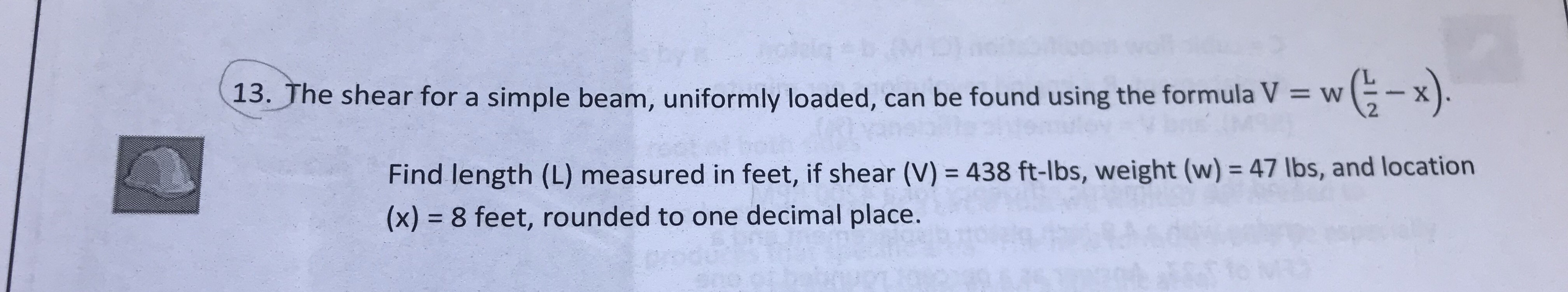(13. The shear for a simple beam, uniformly loaded, can be found using the formula V13. The shear for a simple beam, uniformly loaded, can be found using the formula V xw (-x2Find length (L) measured in feet, if shear (V) 438 ft-lbs, weight (w)- 47 lbs, and location(x) 8 feet, rounded to one decimal place.

Question

Please provide a detailed solutionhelp_outlineImage Transcriptionclose(13. The shear for a simple beam, uniformly loaded, can be found using the formula V 13. The shear for a simple beam, uniformly loaded, can be found using the formula V x w ( -x 2 Find length (L) measured in feet, if shear (V) 438 ft-lbs, weight (w)- 47 lbs, and location (x) 8 feet, rounded to one decimal place. fullscreen
Step 1

Introduction:

Denote the length of a simple beam as L.

Denote the shear of a simple beam as V.

Denote the weight of a simple beam as w.

Denote the location of the simple beam as x.

The general formula to find the shear for a uniformly loaded simple beam is,

Step 2

Obtain the length of the simple beam measured in feet:

The shear of a simple beam is given as V = 438 ft-lbs.

The weight of a simple beam is given as w = 47 lbs.

The location of the simple beam is given as ...

Want to see the full answer?

See Solution

Want to see this answer and more?

Our solutions are written by experts, many with advanced degrees, and available 24/7

See Solution
Tagged in

Other